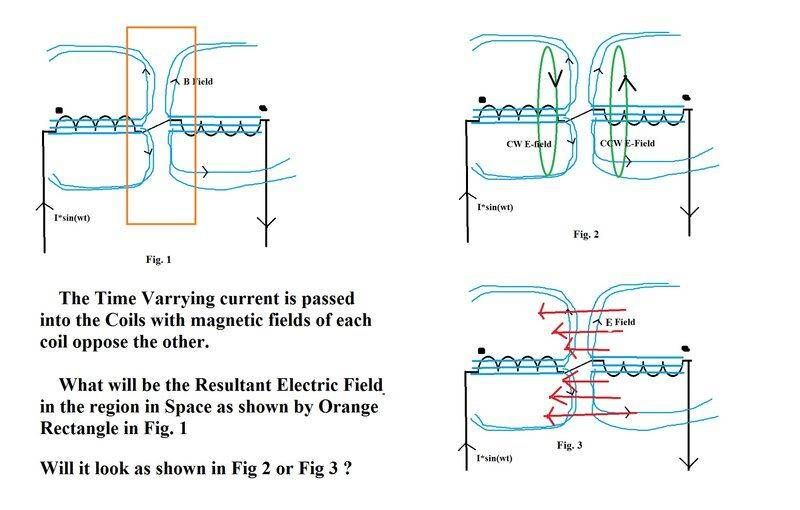# Orientation of Electric Fields in a Bucking Coil Setup

• Narayanan KR

#### Narayanan KR

TL;DR Summary
Imagine two series coils carrying a Time varying current such that magnetic fields of both coils oppose each other, what will be the orientation and polarization of the Induced Electric Fields due to changing magnetic fields in the Space between the coils (circled by Orange Box in Fig. 1) ?
Will it be circular as shown by green arrows in Fig. 2 or will it be axial as shown by red arrows in fig. 3 ?Where is this question from ?
Is this homework ?

Where is this question from ?
Is this homework ?
we do projects for educational purpose, This particular Problem came while working on differential mode choke to reduce noise input in electronics, it would help me a lot if someone answers it.

Will it be circular as shown by green arrows in Fig. 2 or will it be axial as shown by red arrows in fig. 3 ?
I think both electric field distributions described by you will appear. The actual overall electric field distribution may be more complicated. You may need to use 3D electromagnetic simulation software to find accurate answers.

This particular Problem came while working on differential mode choke to reduce noise input in electronics,
For lumped elements like that, the E-field does not enter into the functional analysis. You are only concerned with the currents and the magnetic flux produced by them. You do not need to try to analyze this lumped component like it's an antenna. If you start talking about GHz circuit analysis, that is a different topic, and much more complicated.

We might, perhaps, consider a simple analogy where we have a pair of parallel wires carrying current in the same direction. Then the wires repel each other because the magnetic fields between them are opposing. The electric field of each wire is directed outwards into space like the bristles of a test tube brush, but between the wires the two electric fields are superimposed, and as they act in opposite directions, they cancel to zero.

•Narayanan KR
We might, perhaps, consider a simple analogy where we have a pair of parallel wires carrying current in the same direction. Then the wires repel each other because the magnetic fields between them are opposing. The electric field of each wire is directed outwards into space like the bristles of a test tube brush, but between the wires the two electric fields are superimposed, and as they act in opposite directions, they cancel to zero.
understood

We might, perhaps, consider a simple analogy where we have a pair of parallel wires carrying current in the same direction. Then the wires repel each other because the magnetic fields between them are opposing.
It is easy to get that parallel wire situation backwards in your mind. When a short circuit occurs at the load end of a transmission line, the currents are high, equal, and in opposite directions, so the wires initially repel. When the short is cleared, overhead transmission lines swing back together, where they may contact and so short circuit.
During normal mode operation, the magnetic field between two wires is almost doubled, while the external fields tend to cancel, which is one reason why parallel transmission lines have low radiation.

The OP question is not well posed and so is more complex. The magnetic fields of the two coils will cancel on axis, but will sum away from the axis on the plane of symmetry.

•Narayanan KR
I think he is asking about electric fields, which is complex. Just because they cancel in the centre does not also mean zero coupling.

I think he is asking about electric fields, which is complex. Just because they cancel in the centre does not also mean zero coupling.
So you will not counter or invert the relative current direction in your parallel wire example?

Yes, the Op wants electric field induced in space? about the wires and coils.
If the frequency and inductance were very high, the current would be low, so the electric field due to the supply alone would be important. The magnetic field is needed to find the energy radiation direction, the Poynting vector, and so from that, the electric field component in the complex near field.

•Narayanan KR